Next: Virtual (almost real) photon Up: Incoherent Pair Production Previous: Incoherent Pair Production

### Breit-Wheeler Process

The differential crosssection with respect to the scattering angle of the final electron in the center-of-mass frame is given by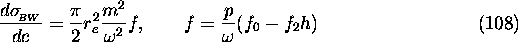with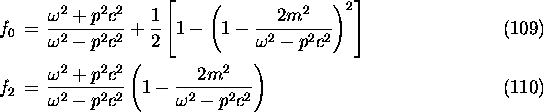where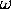, p
Energy and momentum of final electron in the center-of-mass frame.
c
Cosine of the scattering angle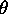of the final electron in the center-of-mass frame.
h
Product of circular polarizations of the two initial photons.

The total crosssection is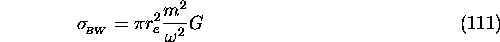where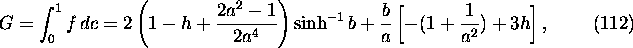where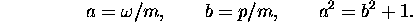Events are generated by the following algorithm using inverse function.

(a)
Compute, p, a, b, G and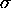for given initial parameters (reject if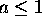, i.e., below threshold) and calculate the event probability for the given time step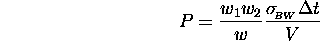where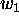and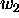are the weights of initial photons (number of real photons divided by that of macro photons), w the weight of the pair to be created,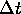the time interval and V the volume in which the macro phtons are located.
(b)
If P is too large (say, >0.1), divide the interval(and P) by an integer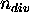, and repeat the following proceduretimes.
(c)
Generate a random number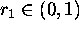. Reject if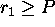.
(d)
Generate another random number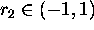and solve the equation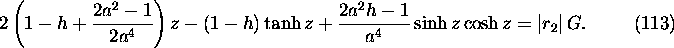with respect to z. Here z is defined by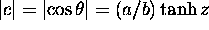(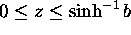). The left hand side is the integral of f from 0 to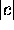. The sign ofis determined by the sign of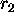.
(e)
Generate another random number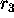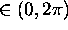and compute the transverse component of electron momentum by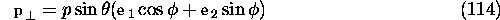where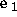and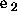are arbitrary unit vectors perpendicular to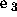, the unit vector along the initial photon momentum in the center-of-mass frame. The latter is given by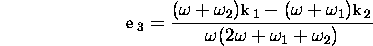where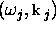are the energy momentum of the photons in the original frame.
The value of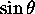should be computed from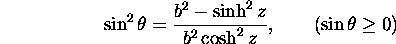rather than from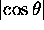because the latter is usually very close to unity whenis much larger than the electron rest mass.
(f)
Then, the momentum of the electron in the original frame is calculated by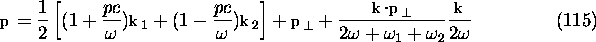where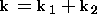. Note that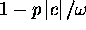must be computed from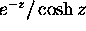in order to avoid round off errors.
(g)
The momentum of positron is computed from the momentum conservation.Next: Virtual (almost real) photon Up: Incoherent Pair Production Previous: Incoherent Pair Production

Toshiaki Tauchi
Thu Dec 3 17:27:26 JST 1998